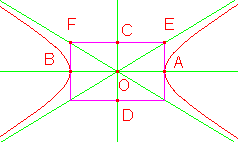www.batmath.it

A Glossary of Mathematical Terms - H

Harmonic mean

Two times the product of two numbers a and b divided by the sum of the numbers.

See Altitude.

See Sphere.

Heptagon

A seven-sided polygon.

Hexagon

A six-sided polygon.

Histogram

A symbolic representation of discrete or continuous data, made using bars, where each bar represents a range of values when the variable is continuous.

HyperbolaOne of the conic sections.
Center: O;
transverse (real) axis: AB;
conjugate axis: CD;
vertices: A, B;
asymptotes: the straight lines OE and OF.

Hypotenuse

The side opposite to the right angle in a right triangle.

first published on september 01 2003 - last updated on september 01 2003